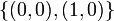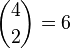Z2 in V4

This article is about a particular subgroup in a group, up to equivalence of subgroups (i.e., an isomorphism of groups that induces the corresponding isomorphism of subgroups). The subgroup is (up to isomorphism) cyclic group:Z2 and the group is (up to isomorphism) Klein four-group (see subgroup structure of Klein four-group).
The subgroup is a normal subgroup and the quotient group is isomorphic to cyclic group:Z2.
VIEW: Group-subgroup pairs with the same subgroup part | Group-subgroup pairs with the same group part| Group-subgroup pairs with the same quotient part | All pages on particular subgroups in groups

Definition

We are interested in the Klein four-group:$G := \{ e,a,b,c \}$

where$e$ is the identity element and$a,b,c$ are all non-identity elements of order 2.

We are interested in the subgroup:$H = H_0 = \{ e, a\}$

as well as the other two subgroups automorphic to$H$:$H_1 = \{ e,b \}, H_2 = \{ e, c \}$

Direct product and direct factor interpretation

This article describes the subgroup$H$ in the group$G$, where$G$ is the Klein four-group:$G := \mathbb{Z}/2\mathbb{Z} \times \mathbb{Z}/2\mathbb{Z}$

Denoted additively, the four elements of$G$ are:$\! \{ (0,0), (0,1), (1,0), (1,1) \}$

where the addition is modulo 2 in each coordinate separately.

and$H = H_0$ is the two-element subgroup:$\! \{ (0,0), (1,0) \}$$H$ is a normal subgroup (since$G$ is an abelian group), so has no other conjugate subgroups. There are two other automorphic subgroups to$H$ in$G$:$\! H_1 = \{ (0,0), (0,1) \}, H_2 = \{ (0,0), (1,1) \}$

The quotient group$G/H$ is isomorphic to cyclic group:Z2.

Cosets

Each of$H$,$H_1$ and$H_2$ is a normal subgroup and each has two cosets: the subgroup itself and the rest of the group. There is a total of six cosets. In fact, every subset of size two in the whole group (total of$\binom{4}{2} = 6$ such subsets) is a coset of exactly one of these three subgroups.

Complements

Any two of the three subgroups$H, H_1, H_2$ are permutable complements of each other. Since they're all normal, this means that$G$ is an internal direct product of any two of them.

Properties related to complementation

Property Meaning Satisfied? Explanation Comment
complemented normal subgroup normal subgroup with permutable complement Yes
complemented characteristic subgroup characteristic subgroup with permutable complement No not a characteristic subgroup
direct factor normal subgroup with normal complement Yes
retract subgroup with normal complement Yes
permutably complemented subgroup subgroup with permutable complement Yes
lattice-complemented subgroup subgroup with lattice complement Yes

Arithmetic functions

Function Value Explanation
order of whole group 4
order of subgroup 2
index 2
size of conjugacy class 1
number of conjugacy classes in automorphism class 3
size of automorphism class 3

Effect of subgroup operators

Function Value as subgroup (descriptive) Value as subgroup (link) Value as group
normalizer the whole group -- Klein four-group
centralizer the whole group -- Klein four-group
normal core the subgroup itself current page cyclic group:Z2
normal closure the subgroup itself current page cyclic group:Z2
characteristic core trivial subgroup -- trivial group
characteristic closure the whole group -- Klein four-group
commutator with whole group trivial subgroup -- trivial group

Subgroup properties

Invariance under automorphisms and endomorphisms: properties

Property Meaning Satisfied? Explanation Comment
normal subgroup invariant under all inner automorphisms, equals its conjugate subgroups Yes abelian implies every subgroup is normal
characteristic subgroup invariant under all automorphisms No Not invariant under automorphism that interchanges coordinates, i.e., that sends$(a,b)$ to$(b,a)$ See also normal not implies characteristic. This is the smallest example of a normal subgroup that is not characteristic and is part of a general family of such examples.
coprime automorphism-invariant subgroup invariant under automorphisms of order coprime to that of the group No The group has an automorphism of order three that cycles the three non-identity elements -- this cyclically permutes the three automorphs of$H$
cofactorial automorphism-invariant subgroup invariant under all automorphisms whose order has no prime factors other than those of the whole group No The coordinate interchange automorphism does not preserve the subgroup, and has order 2
subgroup-cofactorial automorphism-invariant subgroup invariant under all automorphisms whose order has no prime factors other than those of the subgroup No The coordinate interchange automorphism does not preserve the subgroup, and has order 2
retraction-invariant subgroup invariant under all retractions, i.e., endomorphisms that equal their own square No Consider the retraction with kernel$H_1$ and image$H_2$ (realized as projection onto$H_2$ in the realization of$G$ as an internal direct product of$H_1$ and$H_2$). Under this,$H$ maps to$H_2$.

Resemblance-based properties

Property Meaning Satisfied? Explanation Comment
order-isomorphic subgroup isomorphic to any subgroup of the same order Yes follows since order is prime, and there is only one isomorphism class of a group of prime order
order-automorphic subgroup any subgroup of the same order is automorphic to it Yes the three subgroups of order 2 are precisely$H, H_1, H_2$
isomorph-automorphic subgroup any isomorphic subgroup is automorphic Yes the three subgroups of order 2 are precisely$H, H_1, H_2$
isomorph-conjugate subgroup conjugate to every isomorphic subgroup No distinct normal subgroups -- cannot be conjugate
order-conjugate subgroup conjugate to every subgroup of the same order No distinct normal subgroups -- cannot be conjugate
automorph-conjugate subgroup conjugate to every automorphic subgroup No distinct normal subgroups -- cannot be conjugate